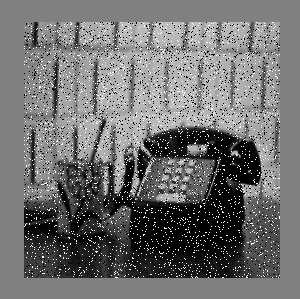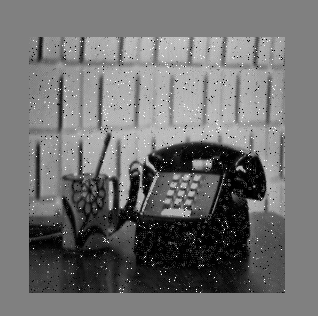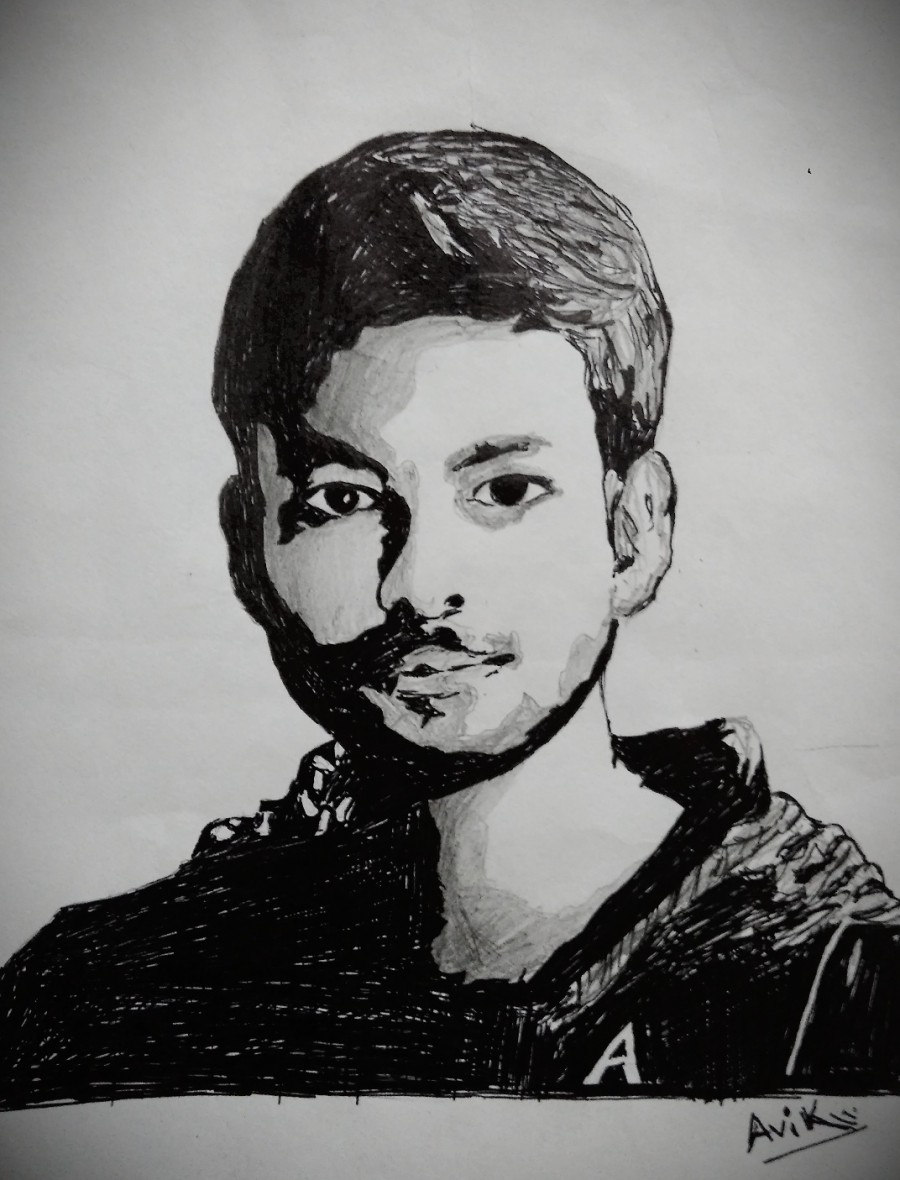# Salt and Pepper Noise removal using C++

Salt-and-pepper noise is a form of noise sometimes seen on images. It is also known as impulse noise. This noise can be caused by sharp and sudden disturbances in the image signal. It presents itself as sparsely occurring white and black pixels. An effective noise reduction method for this type of noise is a median filter or a morphological filter.

In this article, we will learn how to remove Salt-Pepper Noise from the image simply using C++ (without using any external image processing library like OpenCV).

Approach:

1. Store the pixel values of input image in an array.
2. For each pixel value if it contains 0(black) or 255(white) then compute the average value of its neighbor pixel values, else that pixel value is stored in another array.
3. Pixel values of the new array are used for output file.

Below is the simple C++ implementaion of Salt-Pepper Noise removal :

 `#include ` `#include ` `#include ` ` `  `using` `namespace` `std; ` ` `  `int` `array; ``// used for input image ` `int` `arr; ``// used for output image ` `int` `hist; ` ` `  `int` `main() ` `{ ` `    ``int` `i, row = 0, j = 0, col = 0, numrows = 0, numcols = 0, MAX = 0; ` `     `  `    ``// input image ` `    ``ifstream infile(``"saltpepper.pgm"``);  ` `    ``stringstream ss; ` `    ``string inputLine = ``""``; ` ` `  `    ``// First line : version ` `    ``getline(infile, inputLine); ` `    ``if` `(inputLine.compare(``"P2"``) != 0) ` `        ``cerr << ``"Version error"` `<< endl; ` `    ``else` `        ``cout << ``"Version : "` `<< inputLine << endl; ` ` `  `    ``// Continue with a stringstream ` `    ``ss << infile.rdbuf(); ` `     `  `    ``// Secondline : size ` `    ``ss >> numcols >> numrows >> MAX; ` `     `  `    ``// print total number of columns, ` `    ``// rows and maximum intensity of the image ` `    ``cout << numcols << ``" columns and "` `<< numrows << ``" rows"` `<< endl ` `         ``<< ``" Maximium Intesity "` `<< MAX << endl; ` ` `  `    ``// Initialize a new array of ` `    ``// same size of the image with 0 ` `    ``for` `(row = 0; row <= numrows; ++row) ` `        ``array[row] = 0; ` ` `  `    ``for` `(col = 0; col <= numcols; ++col) ` `        ``array[col] = 0; ` ` `  `    ``printf``(``"****\n"``); ` `     `  `    ``// Following lines : data ` `    ``for` `(row = 1; row <= numrows; ++row) { ` `        ``for` `(col = 1; col <= numcols; ++col) { ` `            ``// original data store in new array ` `            ``ss >> array[row][col];  ` `        ``} ` `    ``} ` ` `  `    ``for` `(row = 1; row <= numrows; ++row) { ` `        ``for` `(col = 1; col <= numcols; ++col) { ` `             `  `            ``// check if intensity is black or white ` `            ``if` `(array[row][col] == 0 || array[row][col] == 255)  ` `            ``{ ` `                ``// compute average of neighbours pixels ` `                ``// and store the pixel value in another ` `                ``// array for output image ` `                ``arr[row][col] = (array[row - 1][col] + ` `                                 ``array[row - 1][col - 1] + ` `                                 ``array[row - 1][col + 1] + ` `                                 ``array[row][col - 1] + ` `                                 ``array[row][col + 1] + ` `                                 ``array[row + 1][col + 1] + ` `                                 ``array[row + 1][col] + ` `                                 ``array[row + 1][col - 1]) / 8;  ` `            ``} ` `            ``else` `{ ` `                ``// store pixel value in another ` `                ``// array for output image ` `                ``arr[row][col] = array[row][col];  ` `            ``} ` `        ``} ` `    ``} ` ` `  `    ``ofstream outfile; ` `     `  `    ``// new file open to store the output image ` `    ``outfile.open(``"salt and pepper.pgm"``);  ` `    ``outfile << ``"P2"` `<< endl; ` `    ``outfile << numcols << ``" "` `<< numrows << endl; ` `    ``outfile << ``"255"` `<< endl; ` ` `  `    ``for` `(row = 1; row <= numrows; ++row) { ` `        ``for` `(col = 1; col <= numcols; ++col) { ` `             `  `            ``// store resulatant pixel intensity to the output file ` `            ``outfile << arr[row][col] << ``" "``;  ` `        ``} ` `    ``} ` `     `  `    ``outfile.close(); ` `    ``infile.close(); ` `    ``return` `0; ` `} `

Input Image:Output Image:Rated as one of the most sought after skills in the industry, own the basics of coding with our C++ STL Course and master the very concepts by intense problem-solving.

My Personal Notes arrow_drop_upCheck out this Author's contributed articles.

If you like GeeksforGeeks and would like to contribute, you can also write an article using contribute.geeksforgeeks.org or mail your article to contribute@geeksforgeeks.org. See your article appearing on the GeeksforGeeks main page and help other Geeks.

Please Improve this article if you find anything incorrect by clicking on the "Improve Article" button below.

Article Tags :
Practice Tags :

Be the First to upvote.

Please write to us at contribute@geeksforgeeks.org to report any issue with the above content.